Test: Asymptotic Worst Case Time & Space Complexity- 1

# Test: Asymptotic Worst Case Time & Space Complexity- 1

Test Description

## 20 Questions MCQ Test GATE Computer Science Engineering(CSE) 2023 Mock Test Series | Test: Asymptotic Worst Case Time & Space Complexity- 1

Test: Asymptotic Worst Case Time & Space Complexity- 1 for Computer Science Engineering (CSE) 2023 is part of GATE Computer Science Engineering(CSE) 2023 Mock Test Series preparation. The Test: Asymptotic Worst Case Time & Space Complexity- 1 questions and answers have been prepared according to the Computer Science Engineering (CSE) exam syllabus.The Test: Asymptotic Worst Case Time & Space Complexity- 1 MCQs are made for Computer Science Engineering (CSE) 2023 Exam. Find important definitions, questions, notes, meanings, examples, exercises, MCQs and online tests for Test: Asymptotic Worst Case Time & Space Complexity- 1 below.
Solutions of Test: Asymptotic Worst Case Time & Space Complexity- 1 questions in English are available as part of our GATE Computer Science Engineering(CSE) 2023 Mock Test Series for Computer Science Engineering (CSE) & Test: Asymptotic Worst Case Time & Space Complexity- 1 solutions in Hindi for GATE Computer Science Engineering(CSE) 2023 Mock Test Series course. Download more important topics, notes, lectures and mock test series for Computer Science Engineering (CSE) Exam by signing up for free. Attempt Test: Asymptotic Worst Case Time & Space Complexity- 1 | 20 questions in 60 minutes | Mock test for Computer Science Engineering (CSE) preparation | Free important questions MCQ to study GATE Computer Science Engineering(CSE) 2023 Mock Test Series for Computer Science Engineering (CSE) Exam | Download free PDF with solutions
 1 Crore+ students have signed up on EduRev. Have you?
Test: Asymptotic Worst Case Time & Space Complexity- 1 - Question 1

### What is recurrence for worst case of QuickSort and what is the time complexity in Worst case?

Detailed Solution for Test: Asymptotic Worst Case Time & Space Complexity- 1 - Question 1

The worst case of QuickSort occurs when the picked pivot is always one of the corner elements in sorted array. In worst case, QuickSort recursively calls one subproblem with size 0 and other subproblem with size (n-1). So recurrence is T(n) = T(n-1) + T(0) + O(n) The above expression can be rewritten as T(n) = T(n-1) + O(n) 1

void exchange(int *a, int *b)

{

int temp;

temp = *a;

*a   = *b;

*b   = temp;

}

int partition(int arr[], int si, int ei)

{

int x = arr[ei];

int i = (si - 1);

int j;

for (j = si; j <= ei - 1; j++)

{

if(arr[j] <= x)

{

i++;

exchange(&arr[i], &arr[j]);

}

}

exchange (&arr[i + 1], &arr[ei]);

return (i + 1);

}

/* Implementation of Quick Sort

arr[] --> Array to be sorted

si  --> Starting index

ei  --> Ending index

*/

void quickSort(int arr[], int si, int ei)

{

int pi;    /* Partitioning index */

if(si < ei)

{

pi = partition(arr, si, ei);

quickSort(arr, si, pi - 1);

quickSort(arr, pi + 1, ei);

}

}

Test: Asymptotic Worst Case Time & Space Complexity- 1 - Question 2

### Suppose we have a O(n) time algorithm that finds median of an unsorted array. Now consider a QuickSort implementation where we first find median using the above algorithm, then use median as pivot. What will be the worst case time complexity of this modified QuickSort.

Detailed Solution for Test: Asymptotic Worst Case Time & Space Complexity- 1 - Question 2

If we use median as a pivot element, then the recurrence for all cases becomes T(n) = 2T(n/2) + O(n) The above recurrence can be solved using Master Method. It falls in case 2 of master method.

Test: Asymptotic Worst Case Time & Space Complexity- 1 - Question 3

### Given an unsorted array. The array has this property that every element in array is at most k distance from its position in sorted array where k is a positive integer smaller than size of array. Which sorting algorithm can be easily modified for sorting this array and what is the obtainable time complexity?

Test: Asymptotic Worst Case Time & Space Complexity- 1 - Question 4

Which of the following is not true about comparison based sorting algorithms?

Test: Asymptotic Worst Case Time & Space Complexity- 1 - Question 5

What is time complexity of fun()?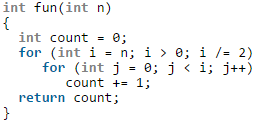Detailed Solution for Test: Asymptotic Worst Case Time & Space Complexity- 1 - Question 5

For a input integer n, the innermost statement of fun() is executed following times. n + n/2 + n/4 + ... 1 So time complexity T(n) can be written as T(n) = O(n + n/2 + n/4 + ... 1) = O(n) The value of count is also n + n/2 + n/4 + .. + 1

Test: Asymptotic Worst Case Time & Space Complexity- 1 - Question 6

What is the time complexity of fun()?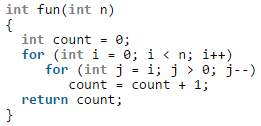Detailed Solution for Test: Asymptotic Worst Case Time & Space Complexity- 1 - Question 6

The time complexity can be calculated by counting number of times the expression "count = count + 1;" is executed. The expression is executed 0 + 1 + 2 + 3 + 4 + .... + (n-1) times. Time complexity =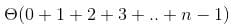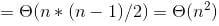Test: Asymptotic Worst Case Time & Space Complexity- 1 - Question 7

The recurrence relation capturing the optimal time of the Tower of Hanoi problem with n discs is.

Detailed Solution for Test: Asymptotic Worst Case Time & Space Complexity- 1 - Question 7

Following are the steps to follow to solve Tower of Hanoi problem recursively.

Let the three pegs be A, B and C. The goal is to move n pegs from A to C. To move n discs from peg A to peg C: move n-1 discs from A to B. This leaves disc n alone on peg A move disc n from A to C move n?1 discs from B to C so they sit on disc n

The recurrence function T(n) for time complexity of the above recursive solution can be written as following. T(n) = 2T(n-1) + 1

Test: Asymptotic Worst Case Time & Space Complexity- 1 - Question 8

Let w(n) and A(n) denote respectively, the worst case and average case running time of an algorithm executed on an input of size n. which of the following is ALWAYS TRUE?

Detailed Solution for Test: Asymptotic Worst Case Time & Space Complexity- 1 - Question 8

The worst case time complexity is always greater than or same as the average case time complexity.

Test: Asymptotic Worst Case Time & Space Complexity- 1 - Question 9

Which of the following is not O(n^2)?

Detailed Solution for Test: Asymptotic Worst Case Time & Space Complexity- 1 - Question 9

The order of growth of option c is n^2.5 which is higher than n^2.

Test: Asymptotic Worst Case Time & Space Complexity- 1 - Question 10

Which of the given options provides the increasing order of asymptotic complexity of functions f1, f2, f3 and f4?

f1(n) = 2^n
f2(n) = n^(3/2)
f3(n) = nLogn
f4(n) = n^(Logn)

Detailed Solution for Test: Asymptotic Worst Case Time & Space Complexity- 1 - Question 10

f1(n) = 2^n
f2(n) = n^(3/2)
f3(n) = nLogn
f4(n) = n^(Logn)

Except f3, all other are exponential. So f3 is definitely first in output. Among remaining, n^(3/2) is next. One way to compare f1 and f4 is to take Log of both functions. Order of growth of Log(f1(n)) is Θ(n) and order of growth of Log(f4(n)) is Θ(Logn * Logn). Since Θ(n) has higher growth than Θ(Logn * Logn), f1(n) grows faster than f4(n). Following is another way to compare f1 and f4. Let us compare f4 and f1. Let us take few values to compare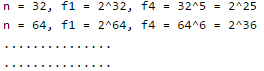Test: Asymptotic Worst Case Time & Space Complexity- 1 - Question 11

Consider the following program fragment for reversing the digits in a given integer to obtain a new integer. Let n = D1D2…Dm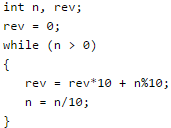Q. The loop invariant condition at the end of the ith iteration is:

Detailed Solution for Test: Asymptotic Worst Case Time & Space Complexity- 1 - Question 11

We can get it by taking an example like n = 54321. After 2 iterations, rev would be 12 and n would be 543.

Test: Asymptotic Worst Case Time & Space Complexity- 1 - Question 12

What is the best time complexity of bubble sort?

Detailed Solution for Test: Asymptotic Worst Case Time & Space Complexity- 1 - Question 12

The bubble sort is at its best if the input data is sorted. i.e. If the input data is sorted in the same order as expected output. This can be achieved by using one boolean variable. The boolean variable is used to check whether the values are swapped at least once in the inner loop. Consider the following code snippet:
[sourcecode language="C"]

int main() {
int arr[] = {10, 20, 30, 40, 50}, i, j, isSwapped;
int n = sizeof(arr)/ sizeof(*arr);
isSwapped = 1; for(i = 0; i < n - 1 && isSwapped; ++i)
{
isSwapped = 0;
for(j = 0; j < n - i - 1; ++j)
if (arr[j] > arr[j + 1])
{
swap(&arr[j], &arr[j + 1]);
isSwapped = 1;
} }
for(i = 0; i < n; ++i)

printf("%d ", arr[i]); return 0;
}
[/sourcecode]
Please observe that in the above code, the outer loop runs only once.

Test: Asymptotic Worst Case Time & Space Complexity- 1 - Question 13

What is the worst case time complexity of insertion sort where position of the data to be inserted is calculated using binary search?

Detailed Solution for Test: Asymptotic Worst Case Time & Space Complexity- 1 - Question 13

Applying binary search to calculate the position of the data to be inserted doesn't reduce the time complexity of insertion sort. This is because insertion of a data at an appropriate position involves two steps: 1. Calculate the position. 2. Shift the data from the position calculated in step #1 one step right to create a gap where the data will be inserted. Using binary search reduces the time complexity in step #1 from O(N) to O(logN). But, the time complexity in step #2 still remains O(N). So, overall complexity remains O(N^2).

Test: Asymptotic Worst Case Time & Space Complexity- 1 - Question 14

The tightest lower bound on the number of comparisons, in the worst case, for comparison-based sorting is of the order of

Detailed Solution for Test: Asymptotic Worst Case Time & Space Complexity- 1 - Question 14

The number of comparisons that a comparison sort algorithm requires increases in proportion to Nlog(N), where N is the number of elements to sort. This bound is asymptotically tight: Given a list of distinct numbers (we can assume this because this is a worst-case analysis), there are N factorial permutations exactly one of which is the list in sorted order. The sort algorithm must gain enough information from the comparisons to identify the correct permutations. If the algorithm always completes after at most f(N) steps, it cannot distinguish more than 2^f(N) cases because the keys are distinct and each comparison has only two possible outcomes. Therefore, 2^f(N) >= N! or equivalently f(N) >= log(N!). Since log(N!) is Omega(NlogN), the answer is NlogN. For more details, read here

Test: Asymptotic Worst Case Time & Space Complexity- 1 - Question 15

In a modified merge sort, the input array is splitted at a position one-third of the length(N) of the array. What is the worst case time complexity of this merge sort?

Detailed Solution for Test: Asymptotic Worst Case Time & Space Complexity- 1 - Question 15

The time complexity is given by: T(N) = T(N/3) + T(2N/3) + N Solving the above recurrence relation gives, T(N) = N(logN base 3/2)

Test: Asymptotic Worst Case Time & Space Complexity- 1 - Question 16

What is the time complexity of the below function?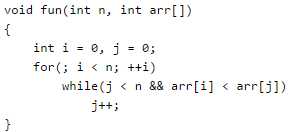Detailed Solution for Test: Asymptotic Worst Case Time & Space Complexity- 1 - Question 16

In the first look, the time complexity seems to be O(n^2) due to two loops. But, please note that the variable j is not initialized for each value of variable i. So, the inner loop runs at most n times. Please observe the difference between the function given in question and the below function:
[sourcecode language="C"]
void fun(int n, int arr[])
{
int i = 0, j = 0;
for(; i < n; ++i)
{
j = 0;
while(j < n && arr[i] < arr[j]) j++;
} }
[/sourcecode]

Test: Asymptotic Worst Case Time & Space Complexity- 1 - Question 17

In a competition, four different functions are observed. All the functions use a single for loop and within the for loop, same set of statements are executed. Consider the following for loops:

Q. If n is the size of input(positive), which function is most efficient(if the task to be performed is not an issue)?

Detailed Solution for Test: Asymptotic Worst Case Time & Space Complexity- 1 - Question 17

The time complexity of first for loop is O(n). The time complexity of second for loop is O(n/2), equivalent to O(n) in asymptotic analysis. The time complexity of third for loop is O(logn). The fourth for loop doesn't terminate.

Test: Asymptotic Worst Case Time & Space Complexity- 1 - Question 18

The following statement is valid. log(n!) = θ(n log n).

Detailed Solution for Test: Asymptotic Worst Case Time & Space Complexity- 1 - Question 18

Order of growth of [Tex]log n![/Tex] and [Tex]nlog n[/Tex] is same for large values of [Tex]n[/Tex], i.e., [Tex] heta (log n!) = heta (nlog n)[/Tex]. So time complexity of fun() is [Tex] heta (nlog n)[/Tex]. The expression [Tex] heta (log n!) = heta (nlog n)[/Tex] can be easily derived from following Stirling's approximation (or Stirling's formula). [Tex] log n! = nlog n - n +O(log(n)) [/Tex]

Test: Asymptotic Worst Case Time & Space Complexity- 1 - Question 19

What does it mean when we say that an algorithm X is asymptotically more efficient than Y?

Detailed Solution for Test: Asymptotic Worst Case Time & Space Complexity- 1 - Question 19

In asymptotic analysis we consider growth of algorithm in terms of input size. An algorithm X is said to be asymptotically better than Y if X takes smaller time than y for all input sizes n larger than a value n0 where n0 > 0.

Test: Asymptotic Worst Case Time & Space Complexity- 1 - Question 20

What is the time complexity of Floyd–Warshall algorithm to calculate all pair shortest path in a graph with n vertices?

Detailed Solution for Test: Asymptotic Worst Case Time & Space Complexity- 1 - Question 20

Floyd–Warshall algorithm uses three nested loops to calculate all pair shortest path. So, time complexity is O(n^3). Read here for more details.

## GATE Computer Science Engineering(CSE) 2023 Mock Test Series

150 docs|215 tests
 Use Code STAYHOME200 and get INR 200 additional OFF Use Coupon Code
Information about Test: Asymptotic Worst Case Time & Space Complexity- 1 Page
In this test you can find the Exam questions for Test: Asymptotic Worst Case Time & Space Complexity- 1 solved & explained in the simplest way possible. Besides giving Questions and answers for Test: Asymptotic Worst Case Time & Space Complexity- 1, EduRev gives you an ample number of Online tests for practice

## GATE Computer Science Engineering(CSE) 2023 Mock Test Series

150 docs|215 tests